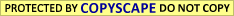# SIMPLE WAY TO FIND OUT TIME IN THE SKY AND BIRTH TIME ( PART 1)

Very  few astrologers actually work out Birth chart by hand ( using paper and pen etc). But it is important to know how to do it. And converting Birth Time to Local Mean Time is the first step. This is because Birth Time is based on Time zone of the country where the birth has taken place  where as Local mean time is based on the exact longitude of place of birth ( not based on time zone of the country).  Now let us forget about Latitudes and focus only on Longitudes. Right!

Suppose some one is born in Banaglore at 2:26 PM.  Bangalore is in India. So the above time is called Indian Standard Time. This is not the birth time to be used for astrological purpose. Because Indian Standard Time is based on Longitude 82 degree 30 minutes East of Greenwich. The longitude of Bangalore is 77 degree 34 minutes East of Greenwich.

Now we if express the Longitudes in Time. Simply divide the degree and minutes by 15, and we get ..

Longitude of Bangalore expressed in Time  5 h 10m 16 s

Indian Standard Longitude expressed in Time 5 h 30 m

( Since the Longitude is East of Greenwich, please put + sign. Thus we get Time zone of India as + 5 h 30 m GMT)

So when it is 12 noon in Greenwich, Time at Bangalore will be 5 h 10 m 16 s PM whilst the clock at this time show 5 h 30 m pm in India.

That means Local Mean Time is based on the Exact Longitude of the place( eg Bangalore) where as Standard Time ( or clock time) is based on the Time zone of the country ( eg India). In other words Local Mean Time is Local. It is based on your exact location.

So Bangalore time is 19m 44 sec (approximately) less than the Indian Standard Time. So please deduct 19m 44 sec from the given Birth Time 2h 26m pm which gives 2 h 6m 16 s pm as Local Mean Time. This is the Time which astrologers used to calculate the Lagna ( Ascendant ) and the cusps of 12 houses etc.